# Rasterio - IO, plotting, histograms

## Python libs for Raster Processing¶¶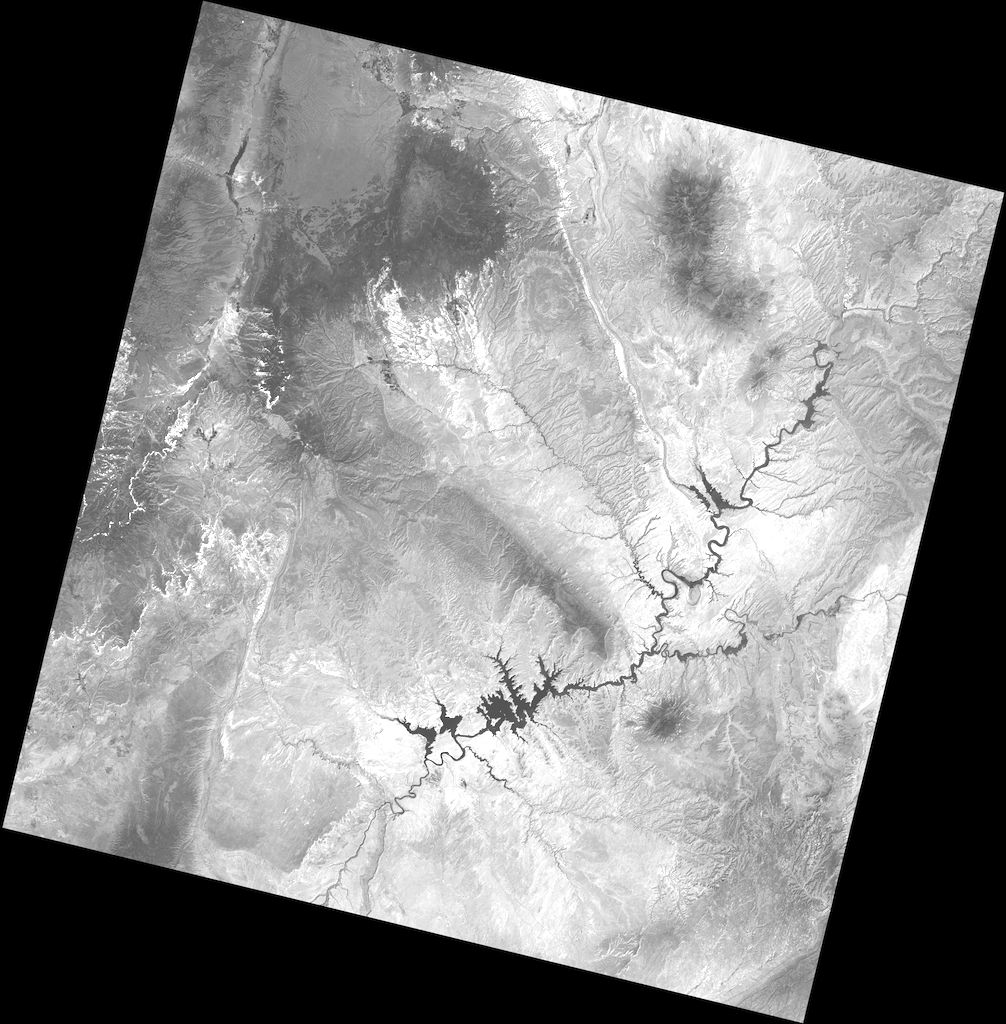The open geospatial Python ecosystem has powerful libraries for processing vector data. We have seen the likes of geopandas, pyshp, shapely, folium, fiona, pysal etc. How about for raster data processing? This notebook explores a few of them, starting with rasterio. My understanding so far is, in general digital image and signal processing via Python is a well established field. Thus if a library allows you to read geospatial image formats into numpy arrays and write the results back, you can easily accomplish your processing using standard Pythonic data analysis process of reading into numpy arrays, doing filtering, enhancements, analysis and presisting into the native file format of choice.

Let us see how far this theory holds up.

In :
45+677

Out:
722

### Accessing raster files¶¶

In :
import rasterio
import matplotlib.pyplot as plt
%matplotlib inline

In :
raster = rasterio.open(path='/Users/atma6951/Documents/GIS_data/Imagery/open-geo/Helsinki_masked_p188r018_7t20020529_z34__LV-FIN.tif')
type(raster)

Out:
rasterio._io.RasterReader

Let us query a few important properties to understand the image better

In :
raster.crs

Out:
CRS({'proj': 'tmerc', 'lat_0': 0, 'lon_0': -183, 'k': 0.9996, 'x_0': 500000, 'y_0': 0, 'datum': 'WGS84', 'units': 'm', 'no_defs': True})
In :
raster.shape

Out:
(1439, 1288)
In :
raster.bounds

Out:
BoundingBox(left=698592.0, bottom=6656859.0, right=735300.0, top=6697870.5)
In :
raster.count

Out:
7
In :
raster.profile

Out:
{'driver': 'GTiff',
'dtype': 'uint8',
'nodata': None,
'width': 1288,
'height': 1439,
'count': 7,
'crs': CRS({'proj': 'tmerc', 'lat_0': 0, 'lon_0': -183, 'k': 0.9996, 'x_0': 500000, 'y_0': 0, 'datum': 'WGS84', 'units': 'm', 'no_defs': True}),
'transform': (698592.0, 28.5, 0.0, 6697870.5, 0.0, -28.5),
'affine': Affine(28.5, 0.0, 698592.0,
0.0, -28.5, 6697870.5),
'tiled': False,
'interleave': 'pixel'}

In :
band1 = raster.read()
type(band1)

Out:
numpy.ndarray
In :
band1.shape, band1.ndim

Out:
((1, 1439, 1288), 3)
In :
band1.min(), band1.max(), band1.mean()

Out:
(0, 255, 59.63132232528628)
##### Read into a specified shape¶¶

I am unable to get this into a 2D array no matter what. Could be a bug

In :
band1a = raster.read(, out_shape=(raster.profile['width'], raster.profile['height']))
band1a.reshape(1288, 1439)
band1a.shape

Out:
(1, 1288, 1439)

#### Preview the band¶¶

In :
band1a

Out:
array([[[0, 0, 0, ..., 0, 0, 0],
[0, 0, 0, ..., 0, 0, 0],
[0, 0, 0, ..., 0, 0, 0],
...,
[0, 0, 0, ..., 0, 0, 0],
[0, 0, 0, ..., 0, 0, 0],
[0, 0, 0, ..., 0, 0, 0]]], dtype=uint8)
In :
from rasterio.plot import show
show(band1a)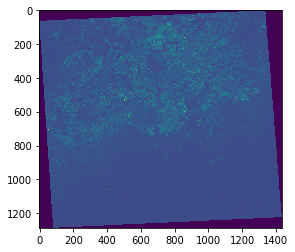Out:
<matplotlib.axes._subplots.AxesSubplot at 0x121ca41d0>

Making a FCC or TCC

In :
fig, ax = plt.subplots(figsize=(8,8))
show(band1a, cmap='Blues', ax=ax, alpha=0.33)

Out:
<matplotlib.axes._subplots.AxesSubplot at 0x10df7fcc0>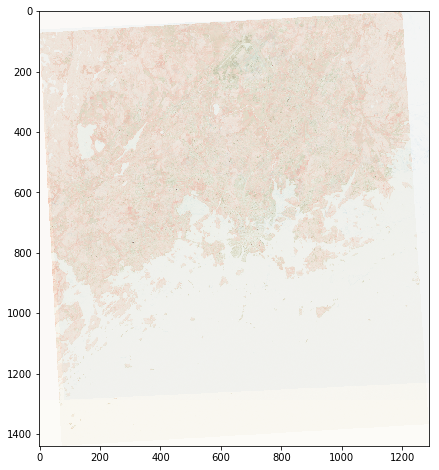#### Plotting histograms¶¶

In :
from rasterio.plot import show_hist
show_hist(source=raster, bins=50, title='Histogram of all bands',
histtype='stepfilled', alpha=0.5)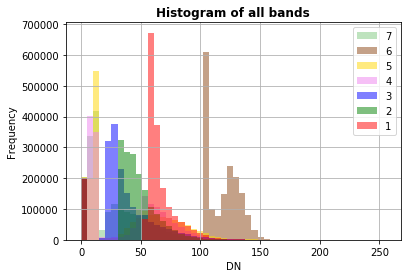#### Read all bands into a 3D array¶¶

In :
all_bands = raster.read()
all_bands.shape

Out:
(7, 1439, 1288)
In :
all_bands.ndim

Out:
3

### Retrieving pixel values¶¶

This landast has 8 bands. Calling the index() method of rasterio._io.RasterReader with spatial coordinates, returns the translation in array indices. You can then use the regular numpy array indexing on the numpy.ndarray object you get as a result of reading the raster image as shown above.

Get pixel values in band 1 at X,Y: (717389, 6675310)

In :
x,y = 717389, 6675310
array_coords = raster.index(x, y)
array_coords

Out:
(791, 659)

This array coordinates is the same for all bands as the dimensions of all bands is the same. Thus you can use regular array indexing as shown below to get the pixel values:

In :
band1[0,791,659]

Out:
83

#### Retrieving pixel values across all bands.¶¶

Let us define a function that returns the pixel values across all bands

In :
[all_bands[i,array_coords, array_coords] for
i in range(raster.count)]

Out:
[83, 62, 66, 32, 53, 139, 48]
In :
def get_cell_value(raster, all_bands, x,y):
array_coords = raster.index(x,y)
n_bands = raster.count

# get pixel values
values= [all_bands[i, array_coords, array_coords]
for i in range(raster.count)]

# plot values
plt.plot(values)
return values


You can use this to construct spectral profiles of various points.

In :
get_cell_value(raster, all_bands, 702829, 6685820)

Out:
[68, 50, 42, 12, 12, 116, 11]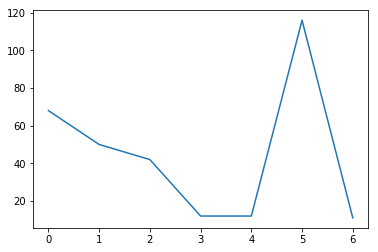In :
get_cell_value(raster, all_bands, 713891, 6688037)

Out:
[69, 60, 47, 102, 87, 133, 42]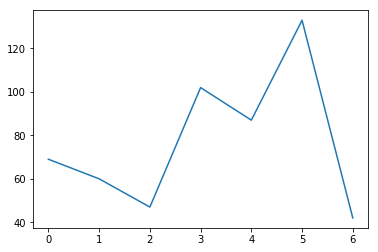Comparing this with QGIS, the values and charts are consistent.